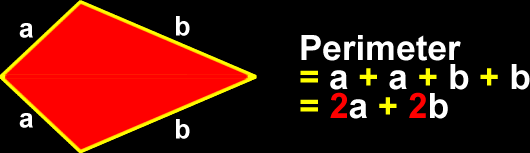# The Properties of a Kite

Definitions and formulas for the perimeter of a kite, the area of a kite, properties of the sides and angles of a kite
Just scroll down or click on what you want and I'll scroll down for you!

 perimeter of a kite area of a kite properties of thesides and angles of a kite properties of the diagonals of a kite

 The perimeter of a kite: To find the perimeter of a kite, just add up all the lengths of the sides:The area of a kite: To find the area of a kite, multiply the lengths of the two diagonals and divide by 2 (same as multiplying by 1/2):The sides and angles of a kite:There are two sets of adjacent sides (next to each other) that are the same length (congruent.) There is one set of congruent angles.  These are opposite of each other and are between sides that are different lengths. On this one, it's kind of hard to put this stuff into words that aren't confusing...  So, look at the picture!